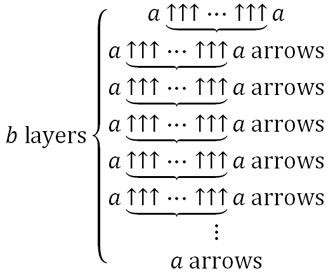Not to be confused with Expansion rule.Visualization of $$a \{\{1\}\} b$$ using Knuth's up arrow notation.

Expansion refers to the binary function $$a \{\{1\}\} b = a \{a \{\cdots \{a\} \cdots\}a\}a$$, where there are b a's from the center out. It is $$\{a,b,1,2\}$$ in BEAF and a{X+1}b in X-Sequence Hyper-Exponential Notation. The notation a{c}b means {a,b,c}, which is a "c + 2"-ated to b, using the bracket operator.

The function eventually dominates any hyper-operator, such as tetration, pentation, or even centation, as well as nested hyper-operators.

Graham's number is defined using a very close variant of expansion. It is $$3 \{\{1\}\} 65$$ with the central 3 replaced with a 4.

$$a\{\{1\}\}b$$ is exactly equal to [a,a,a,b-1] in the Graham Array Notation.

By Bird's Proof, $$a \{\{1\}\} b > a \rightarrow a \rightarrow (b-1) \rightarrow 2$$ using chained arrow notation.

## Examples

• $$2\ \{\{1\}\}\ 2$$ = 4
• $$2\ \{\{1\}\}\ 3 = 2\{2\{2\}2\}2 = 2\{4\}2 = 2\{3\}2 = 2\{2\}2 = 2\{1\}2 = 4$$
• In fact, if the base is equal to 2 and prime is ≥ 2, then the result will always be 4.
• $$3\ \{\{1\}\}\ 2 = \{3,2,1,2\} = 3 \{3\} 3 = 3\uparrow\uparrow\uparrow 3$$ (tritri)
• $$a\ \{\{1\}\}\ 2 = \{a,2,1,2\} = a \{a\} a = a\underbrace{\uparrow\uparrow\cdots\uparrow\uparrow}_{a}a$$
• $$3\ \{\{1\}\}\ 3 = \{3,3,1,2\} = 3 \{3 \{3\} 3\} 3 = 3\{\text{tritri}\}3 = 3\underbrace{\uparrow\uparrow\cdots\uparrow\uparrow}_{\text{tritri}}3$$
• $$4\ \{\{1\}\}\ 3 = \{4,3,1,2\} = 4 \{4 \{4\} 4 \}4 = 4 \{$$$$\text{tritet}$$$$\} 4 = 4\underbrace{\uparrow\uparrow\cdots\uparrow\uparrow}_{\text{tritet}}4$$
• $$a\ \{\{1\}\}\ 3 = \{a,3,1,2\} = a \{a \{a\} a\} a = a\underbrace{\uparrow\uparrow\cdots\uparrow\uparrow}_{a\{a\}a}a$$
• $$3\ \{\{1\}\}\ 4 = \{3,4,1,2\} = 3 \{3 \{3 \{3\} 3\} 3 \}3 = 3 \{3 \{\text{tritri}\} 3\} 3 = 3\{\ \{3,3,1,2\}\ \}3 = 3\underbrace{\uparrow\uparrow\cdots\uparrow\uparrow}_{\{3,3,1,2\} }3$$ = $$3 \uparrow^{3 \uparrow^{3 \uparrow^{3}3}3}3$$
• $$a\ \{\{1\}\}\ 4 = \{a,4,1,2\} = a \{a \{a \{a\} a\} a \}a = a\underbrace{\uparrow\uparrow\cdots\uparrow\uparrow}_{a\{a\{a\}a\}a}a$$
• $$10 \{\{1\}\} 100 = \{10,100,1,2\} = \{10,10,\{10,99,1,2 \}\} = 10 \underbrace{\uparrow\uparrow\cdots\uparrow\uparrow}_{\{10,99,1,2 \} }10$$ (corporal)
• $$a \{\{1\}\} b = \{a,b,1,2\} = \{a,a,\{a,b-1,1,2 \}\} = a \underbrace{\uparrow\uparrow\cdots\uparrow\uparrow}_{\{a,b-1,1,2\} }a$$

## Pseudocode

Below is an example of pseudocode for expansion.

function expansion(a, b):
result := a
repeat b - 1 times:
result := hyper(a, a, result + 2)
return result

function hyper(a, b, n):
if n = 1:
return a + b
result := a
repeat b - 1 times:
result := hyper(a, result, n - 1)
return result


## Approximations in other notations

Notation Approximation
Hyper-E notation $$\textrm Ea\#\#a\#b$$
Chained arrow notation $$a \rightarrow a \rightarrow b \rightarrow 2$$
X-Sequence Hyper-Exponential Notation $$a \{X+1\} b$$ (exact)
Fast-growing hierarchy $$f_{\omega+1}(b)$$
Hardy hierarchy $$H_{\omega^{\omega+1}}(b)$$
Slow-growing hierarchy $$g_{\Gamma_0}(b)$$